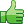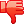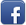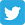Posted on 16th Feb 2014 07:03 pm by admin

Lets see if I can get some help on this one. Can anyone show me what I am doing wrong here. I'm just trying at this point to verify that the form inputs will work right. \$max_img is set to 6. Here is the code:

Code: <form enctype='multipart/form-data' name='inputform' method='post' action='".FUSION_SELF."?op=Add'>

for(\$i=1; \$i<=\$max_img; \$i++){
echo "<tr><td>".\$locale['CLS_0103']."\$i:</td><td>
<input class='textbox' type='file' name='image[]' size='45' maxlength='150' value='http://'></td></tr>";
}

</form>

///////////////////////

\$array = (isset(\$_POST['image']));
\$list = implode(",",\$array);
reset(\$list_array);
foreach(\$list_array AS \$picture) {

echo \$picture."<br />";

}

die;
After 'die' executes I get no output

21512##### Other forums

Count on multidimensional array
Hi, i got an array as below:

Array
(
=> Array
(

Problem!
I have another problem, when i run this code i get this error "Parse error: syntax error, unexp

php email checker
I am having alot of trouble finding info on how to do these email things. I need to do the following

Select Rows as Columns..
is there a way to select COLUMN_NAME from user_tab_columns where table_name='TABLENAME';
and ha

Using real time in php
I'm very average at PHP and im looking to introduce time to something on my site.
Its a sports si